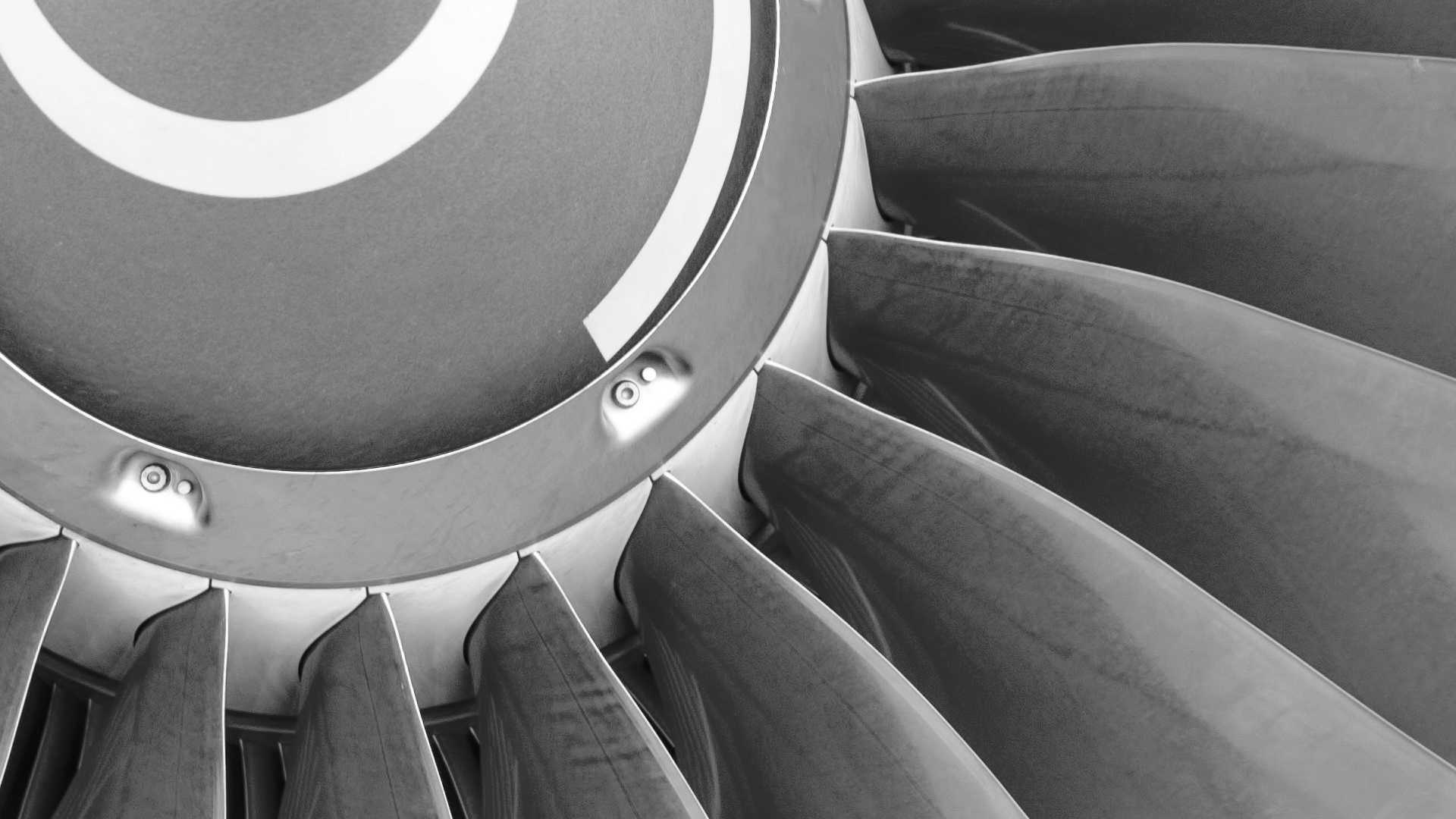Slide 1 - copy - copy# Systems

## System Performance

In general, fans are selected for their ability to deliver a required air volume flow rate against an estimated system resistance to flow, or pressure. This defines an Operating Point and is usually expressed in m³/h at Pascals, or cfm at inches Water Gauge.

## Volume Flow Rate

Volume flow rate is calculated from application data and may be based on a requirement to heat, cool, or ventilate air, ensure even air temperature distribution, or to contain air that has been contaminated.

Where building ventilation is concerned there is a need to extract stale air, fumes and heat at a rate that depends on the purpose of the building. The calculation is based on air changes per hour and may be as few as 1 for a church or 60 for a furnace room.

When extracting a known amount of heat from, for example, a power electronic enclosure, the minimum volume flow rate for a given permitted temperature rise may be calculated using a formula that takes account of the density and specific heat of air.

For a laboratory fume cupboard, or hood, the flow rate is calculated from knowledge of the minimum air speed required to contain fumes at the open sash of a fume cupboard, or through the inlet area of a hood.

## Pressure

Pressure loss is the resistance to air flow and is caused by molecular friction and surface friction as air passes through a system. It is expressed in Pascals (Newtons/m²) or inches Water Gauge and varies with the square of the air velocity. Thus, doubling the flow rate through a given system has the effect of quadrupling the pressure, and halving it quarters the pressure.

The pressure loss within equipment containing complex air paths is difficult to estimate, so requires experience of similar systems. For ductwork, it is a well-researched subject with pressure charts and loss factors for each element in the system.

Static Pressure is the pressure exerted on duct walls and corresponds to potential energy. Velocity Pressure is the flow pressure and corresponds to kinetic energy. The sum of the two is Total Pressure and corresponds to the total energy increase provided by the fan.

Static Pressure loss is estimated by calculating the Velocity Pressure through each component in the ductwork system and multiplying it by a constant, known as a ‘k’ factor for that component, to provide an estimate of the component Static Pressure. The System Static Pressure is then the sum of all component Static Pressures, and the Velocity Pressure is calculated from the discharge air velocity.

By knowing the System Operating Point, it is possible to plot a System Characteristic curve from which the performance of any fan on that system can be estimated.

## Useful Formulae:

Conversion Factors

1 cfm = 1.7m³/h

1litre/s = 3.6m³/h

1m³/s = 3600m³/h

1 mmWG = 9.81Pascals

1inWG = 249Pascals

1mBar = 100Pascals

Note: 1Pascal = 1N/m³

Heat Extraction

Volume flow rate m³/h = (3000 x W)/Δt

W = Power Loss (kW)

Δt = Temp. Rise (K) from inlet to discharge

W = C x A x T

W = Power Loss

C = Coefficient of heat transfer (6 for steel)

A = Surface area of enclosure

T = Temp. difference in/out

Fume Cupboard Air Velocities

(Very general guide. Observe the specification)

At sash test height > 0.50m/s Sash test height = 500mm

In-duct ≈ 6m/s

At stack discharge ≈ 10m/s

Fan Laws

V ∝ N P ∝ N² K ∝ N³ P ∝ I K ∝ I

V = Air Volume Flow Rate

N = Rotational Speed

P = Pressure

K = Absorbed Power

I = Air Density

Pressure

Pv(Pascals) = 1/2pv² or 0.6v²

For Air P= 1.2kg/m³ & V= velocity(m/s)

General Ventilation:

Recommended Air Changes per hour for location:-

Storage = 2

Library = 3

Lab = 5

Bar = 7

Workshop = 8

Café = 10

Kitchen = 25

Paint Shop = 50Slide 1
Need Help?

Our team of Sales Engineers are ready to answer any questions that you may have.Slide 1 - copy - copy
Request a
Brochure

If you would like to receive a brochure listing all of the products on our website, please click below.

## Our Details

Moduflow is the trading name of

Moduflow Fan Systems Ltd,

Registered in England

Company No.04563686